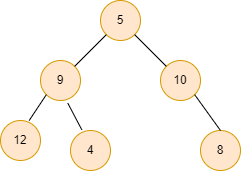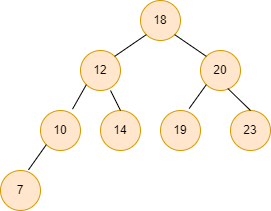# Print the longest path from the root to leaf in a Binary tree

## Introduction

### Problem statement

We are given a Binary tree. Our goal is to print the longest path from the root node to the leaf node in a Binary tree. If there are multiple paths, print any one of them.

### Sample Examples

Example 1:Output:

5 -> 9 -> 12

Explanation:

Longest paths from the root node to the leaf node are:

5 -> 9 -> 12

5 -> 9 -> 4

5 -> 10 -> 8

We can print any of them.

Example 2:Output:

18 -> 12 -> 10 -> 7

## Naive Approach

In this approach, we will generate all the possible paths from the root node to all leaf nodes in the binary tree. We will keep track of the maximum length path and finally print it.

Time complexity: O(n^2)

## Efficient Approach

In this approach, we will be using recursion to solve the problem.

First, we will get the longest path from the left and right subtrees recursively. Then, we will add the root node to one with the longer path.

Thus, we will get the longest path from the root node to the leaf node.

### Steps of algorithm

• If the given root node is null then no path of the binary tree exists, hence we will return an empty vector.
• We will get the longest path from the left subtree in leftvector by recursively traversing root -> left.
• Similarly, we will get the longest path from the right subtree in rightvector by recursively traversing root -> right.
• In the end, we will compare both the sizes of the rightvector and the leftvector. Then, we will add the root node to the longer size and return that particular vector.
• Print the vector in reverse order as we need the longest path from the root to the leaf node.

### Implementation in C++

``````#include <bits/stdc++.h>
using namespace std;

struct Node {
int value;
Node *left;
Node  *right;
};

struct Node* newNode(int value)
{
struct Node* node = new Node;
node->value = value;
node->left = node->right = NULL;

return (node);
}

// Function to get and return the longest path
vector<int> longestPath(Node* root)
{

// If root node is null, no binary tree exists so we return an empty vector
if (root == NULL) {
vector<int> temp = {};
return temp;
}

// Recursive call on left subtree
vector<int> leftvector = longestPath(root->left);

// Recursive call on right subtree
vector<int> rightvector = longestPath(root->right);

// Compare the sizes of the two vectors and add current node

if (rightvector.size() > leftvector.size())
rightvector.push_back(root->value);

else
leftvector.push_back(root->value);

// Return the vector
return (leftvector.size() > rightvector.size() ? leftvector : rightvector);
}

int main()
{
struct Node* root = newNode(1);
root->left = newNode(2);
root->right = newNode(3);
root->left->right = newNode(4);
root->left->left = newNode(5);
root->left->right->left = newNode(6);
root->left->right->left->right = newNode(7);

vector<int> output = longestPath(root);
int n = output.size();

cout << output[n - 1];
for (int i = n - 2; i >= 0; i--) {
cout << " -> " << output[i];
}

return 0;
}``````

Output:

``1 -> 2 -> 4 -> 6 -> 7``

#### Complexity Analysis

Time complexity: O(n), where n is the number of nodes in the given tree as we are traversing all the nodes.

Space complexity: O(h), where h is the height of the given binary tree.

## FAQs

1. Is it possible for a binary tree to have only one child?
A binary tree is a tree in which no node has more than two children, and each child is either a right or left child, even if it is the parent's only child. A full binary tree is the one with two children for each internal node.

2. In a binary tree, how do you count the number of nodes?
Return the value of (2^height – 1) as the resultant count of nodes if the left and right heights of the given Tree are equal to the current root value. Otherwise, call the function for the left and right subtrees recursively and return the sum + 1 as the resultant node count.

3. How to recognise a leaf node in a binary tree?
Steps in C++:
- If root==NULL, return.
- If root->left==NULL and root->right==NULL, it is a leaf node, print the node data.
- Repeat the process for both the left and the right subtree.

## Key Takeaways

This article discussed the approach to print the longest path from the root to leaf node in a Binary tree with examples for better understanding the problem and its C++ code.

If you are interested in coding and want to learn DSA, you can check our guided path for DSA, which is absolutely free!